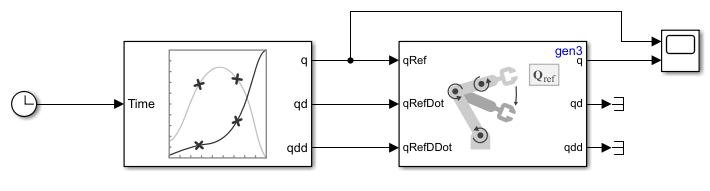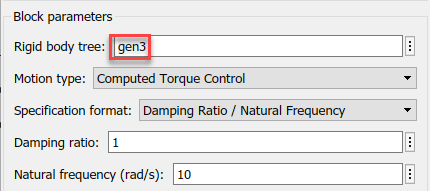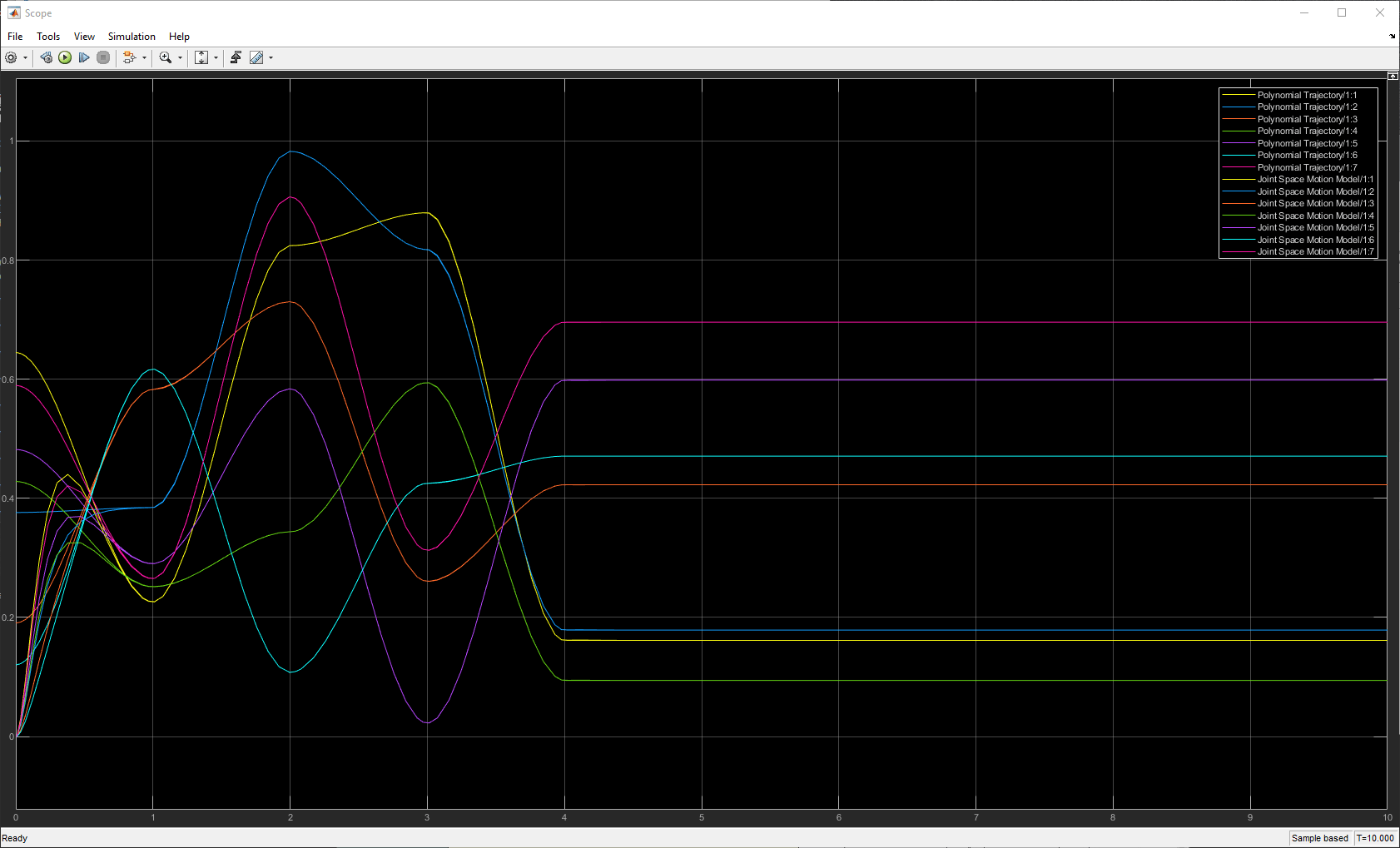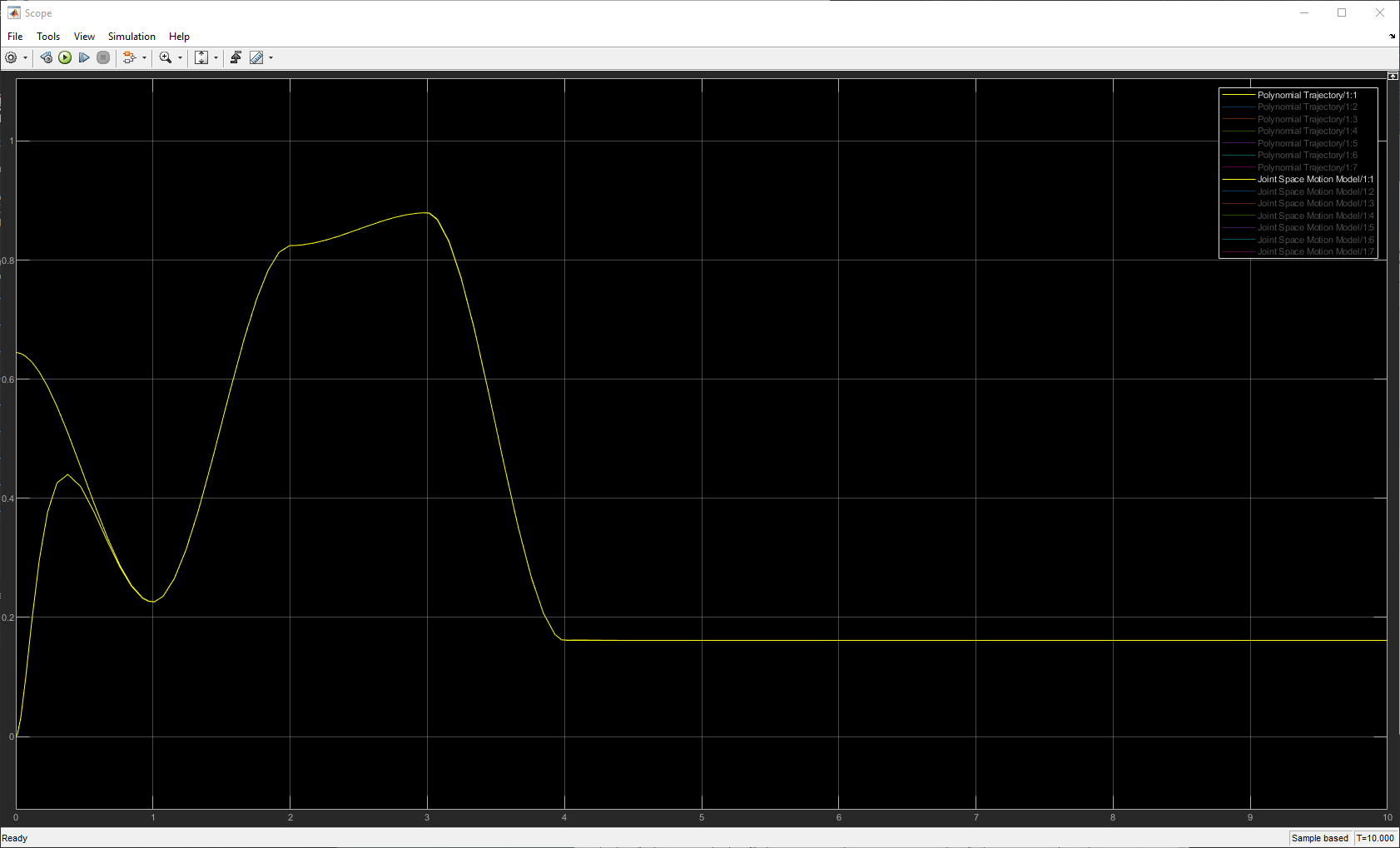# Follow Joint Space Trajectory in Simulink

This example shows how to use a Joint Space Motion Model block to follow a trajectory in Simulink.

This example uses the Kinova Gen3 manipulator robot to follow the trajectories. Load the Gen3 manipulator using `loadrobot` and save the `RigidBodyTree` output as `gen3`. Open the Simulink model.

`[gen3,metadata] = loadrobot("kinovaGen3");`

Open the simulink model.

`open_system("followJointSpaceTrajectoryModel.slx");`### Plan Trajectory

The Polynomial Trajectory block generates a trajectory from a set of waypoints specified in the Waypoints parameter in joint space. This example uses five time points, specified row vector and also the Kinova Gen3 has seven degrees of freedom, so the waypoints matrix must be a 7-by-5 size matrix. The block is set up to generate a new set of waypoints every simulation.

### Motion Model

The Joint Space Motion Model uses a RigidBodyTree, `gen3`, to calculate the joint positions to reach the random trajectory generated by the Polynomial Trajectory block. Leave the other block parameters as default.### Visualize Results

The joint target positions and the calculated joint values from the Joint Space Motion Model connect to a Scope block. Using the legend, you can select a smaller set of signals to compare with better clarity.Observe that the signals for the first joint start separated, and overlap when time is equal to `1`s. So from the initial configuration, the first joint was able to follow the trajectory.